## Wednesday, November 10, 2021

### FFT Parabolic Interpolation

Someone at the Numerix-DSP blog made the reasonable suggestion of interpolating the results of an FFT using a parabola. I did some experimenting with this method and found that it was not any worse than my other methods and it seemed less likely to turn out spurious results.

I've moved out the Maxima domain with my calculations and into Common Lisp, but to create the calculation for the parabolic interpolation, Maxima was of some help. First, we define a function for taking three points, and producing the parabola that is defined by that.

Then we will solve for it. We make the observation that the shape of the parabola is the same regardless of shifting it left or right along the x-axis (or index axis, which corresponds to the frequency axis when we multiply by sampling rate). This being the case, we have no need to supply the final indices but can consider our middle point as index 0, the point before it as index -1 and the point after it as index 1. This simplifies the calculation so that we can find the location of the peak relative to the index we are examining and apply the results of this calculation to it. Time to pull in some grade 12 math and differentiate that parabola and find the vertex (see Fig. 1).Fig. 1. The green dot is approximately where the vertex of this parabola is. The result of this calculation would have x as slightly positive.
$\frac{{{x}^{2}}\, \left( \mathit{y2}-2 \mathit{y1}+\mathit{y0}\right) }{2}-\frac{x\, \left( \mathit{y0}-\mathit{y2}\right) }{2}+\mathit{y1}=y$ $\frac{\left( {{x}^{2}}+x\right) \, \mathit{y2}+\left( 2-2 {{x}^{2}}\right) \, \mathit{y1}+\left( {{x}^{2}}-x\right) \, \mathit{y0}}{2}=y$ $x\, \left( \mathit{y2}-2 \mathit{y1}+\mathit{y0}\right) -\frac{\mathit{y0}-\mathit{y2}}{2}=0$ $[x=-\frac{\mathit{y2}-\mathit{y0}}{2 \mathit{y2}-4 \mathit{y1}+2 \mathit{y0}}]$

On the basis of this we can produce a common lisp function to perform this calculation.

Note that if the peak is to the left of our high point, we will get a negative x value and if it is to the right, a positive value. It will be on the interval $$(-1, 1)$$. Some code that goes through the results of an FFT and takes the absolute value as in our previous work and finds local peaks is all we need now. Where there is a local peak of consecutive absolute values $$a$$, $$b$$, and $$c$$ such that $$a, c < b$$, do some interpolation using parabola-peak-frequency to get an interpolated amplitude and an index adjustment for the frequency index. Then the calculation for the the frequency becomes

$(o + x) s/N$

where o is the index of $$b$$, x is the result from parabola-peak-frequency, s is the sample rate, and $$N$$is the length of elements in the FFT.

Now, maybe we can start applying some math to some real world problems, like, um, Mozart's Piano Concerto No. 13, movement II? Maybe just a snatch within the first second of it for now.Fig. 2. Mozart, anyone?

A general outline on how to proceed with this is to identify all of the local peaks, take the top K highest amplitude data points, interpolate those using the parabolic interpolation above, apply pitch correction (if desired) and midi note calculation. This doesn't address determining pitch durations. Another interesting problem is to try to guess which lower peaks are harmonics of lower pitches. These, and probably other confounding problems, yet await.

## Thursday, September 2, 2021

### SQLite Bulk Insert

I was trying to insert a lot of records into an SQLite database and it was taking a long time. Lots amounted to over 137000 in one table. It was taking hours to complete. I decided it must be possible to do the insertion faster with some dynamic SQL. The approach was simple and probably lends itself to some basic abstraction, but I didn't go that far. I could probably use reflection to remove some of the icky repetitive code in it, but this was ok for my immediate needs.

First up, a basic abstract class to inherit. I include some basic convenience functions in it although it might not be great style.

Next up, inherit the class in the table definitions you will use.

Here are the two main reuseable routines that save a lot of time doing the inserts. They assume all the records are really of the same type, which is the most common application for a bulk insert. I don't know of any reason to make the inserts accommodate multiple types, but it would be possible to apply a partition at the start if you have an application for that.

Note that the syntax for these inserts is very specific to SQLite.

## Saturday, May 22, 2021

### What's in Your Vocabulary Deck?

I am something of a student of New Testament Greek and made my beginnings a few years ago with Mounce's Basics of Biblical Greek. Along with the text book was a flash card program called FlashWorks, and it is a solid way to learn your vocab. The vocab was indexed with frequency and chapter and user level difficulty (based on how often you answered correctly) which was almost all that I wanted to help me keep track of which words I should be working on.

There was one thing that I felt was missing, though I wasn't sure how to define it or to decide what could be done about it. Something like, "how do I get a balanced randomness to my vocab selection if I only want to do a few right now?"

I don't want to randomly select 10 words from out of 500 words. I won't get much repetition if I do that every day. On the other hand, if I only focus on the most frequent, hard words, then I repeat them every day, then the risk might be that I "cram" them and don't really get them into long term memory. So, maybe the thing to do is to start with a selection of 30 words that are the most difficult/frequent words and randomly select 10 from that list. I call it a "pool factor". The pool factor in that case, would be 3. Make a selection of the most difficult, frequent words up to 30 long (and include a random sorting factor in case there are contenders for the last spots in the 30) and then randomly select 10 out of the 30.

The 30 words are a kind of "working set" that I am working toward mastering, although, I don't need to specifically decide on them, they get selected based on criteria. I do 10 words today out of those 30. As I do the daily work, some of those 30 words drop out because I got them right a bunch of time and new words enter the selection of 30.

The next issue is to decide what is meant by hardest, frequent words. If I use a score that starts high for words that have never been marked correct, then I can do a descending sort on score, then by frequency. The whole deck will start at the highest score and initially I am getting the most frequent words. In order to prevent words from disappearing from this set too soon, keep track of not just a score, but the number of times marked correct. Only decrease the score after after getting the word right correct, say, 3 consecutive times. (Since the score of a word doesn't change until you have reached a level of mastery with it, you don't run into the scenario of interchanging sets of words that you then never master.)

Note that you can probably apply this strategy to other types of vocabulary that don't reference a fixed body of literature, but you have to come up with some kind of importance rating on each word in your database that serves the same purpose as the frequency field here.

The code below belongs to a C# project of mine that is using SQLiteAsyncConnection with some fields that are still missing data (hence, ifnull):

public Task<List<Vocab>> GetNRandomHardFrequent(int numberOfWords, double poolFactor)
{
int PoolSize = Convert.ToInt32(numberOfWords * poolFactor);
Random rand = new Random();

string query = @"
select
sub.*
from
(select
v.*
from
Vocab v
order by
ifnull(v.score, 0) desc, ifnull(v.frequency, 0) desc, RANDOM()
limit ?) sub
order by
RANDOM()
limit ?;";

var words = database.QueryAsync<Vocab>(query, PoolSize, numberOfWords);

return words;
}

## Saturday, March 27, 2021

### FFT - DIY

There's more than enough information freely available to enable you to make your own FFT. You can review the pseudo-code from Wikipedia to make it yourself. I have been raving about this bit of Mathematics for several posts now. I knew that the FFT ($$O(N \log {N})$$) was much faster than computing the DFT ($$O(N^2)$$) from the definition. But how much faster is it really when you compare the methods with realistic data sets? Sorting algorithms have this difference but with small data sets (even thousands of points) like most of us work with, it doesn't matter that much.

Whenever you go about to make something that is somewhat complex, you want a more than casual check to verify that you have the right answer. One way of doing this is to create a simple method that doesn't have any complexities related to speeding up the algorithm or computation in it and use it as a reference against the more complex method you want to test. You can also find the results of someone else's work and compare against that.

I made both a DFT and FFT (dit-fft2) method and tried them out. I noticed while testing these out that I get results that agree with each other, but they differ from the results I got in Maxima in two ways: 1) the overall values appear to be factored in the Maxima FFT and there was a difference in sign on the imaginary component. I suspect Maxima is normalizing by length and using positive roots of unity instead of negative roots of unity. (Signal processing people like negative roots of unity and some other people like positive roots of unity. Go figure.) To test for consistency I used create-test-data for a small data set of 32 points and applied both dft and dit-fft2 to it. They come out the same, so I'm reasonably certain that the calculation was done correctly.

Next, I tried a larger data set, $$2^{14}$$ data points. With a sampling rate of 44100 Hz, this is about 0.37s worth of PCM data. The DFT function ran in 2:41 min and the dit-fft2 function ran in 0.125s. Even at 0.125s, that is a long time to process if you have much data to churn through. A brief review of the dit-fft2 function will show that there is a lot of data copying involved in this version and so methods which avoid the need to copy data around by using an indexing scheme are likely to increase performance significantly.

## Monday, March 22, 2021

### FFT - Revisiting Symmetry

There was something missing in my last post that might have created part of the problem with the results. Although I observe what looked like symmetry, I didn't constrain any of my interpolated results based on that assumption of symmetry. So, I thought to myself, there ought to be a mathematical way to tell my equations that they should assume a symmetrical situation, the way I am expecting it to look. I'm not really sure this is broadly applicable, but my intuition about a sine wave is that it should have some kind of symmetry in the resulting appearance. This seems to be borne out by scattered examples of continuous Fourier transforms.

I'm not yet sure how to use symmetry, to find the value, but I can see a way to evaluate the accuracy of a given guess. Mind you, I only know how to evaluate it by appearance. Let's introduce a little formula I will call the flip formula.

$$F(x, C) = 2 C - x$$

Suppose you choose $$C = 440$$, meaning that you are going to treat 440 as the center. So, for example, $$F(439, 440) = 441$$ and $$F(441, 440) = 439$$. Let's apply the flip function to the data in one of our previous examples and see what it looks like when we assume the center is 440.

First, here is the graph we had with just a few points near the peak: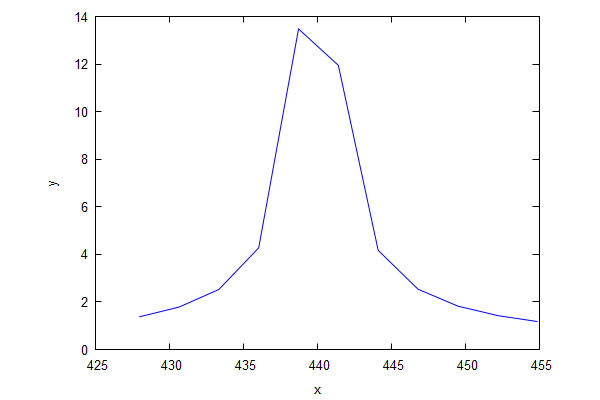Fig. 1. Just the results of the FFT.

Let's have a look at what it looks like when we use 439.5, 439.8, and 440.Fig. 2. "Guessing" frequency 439.5 Hz. Sure doesn't look smooth.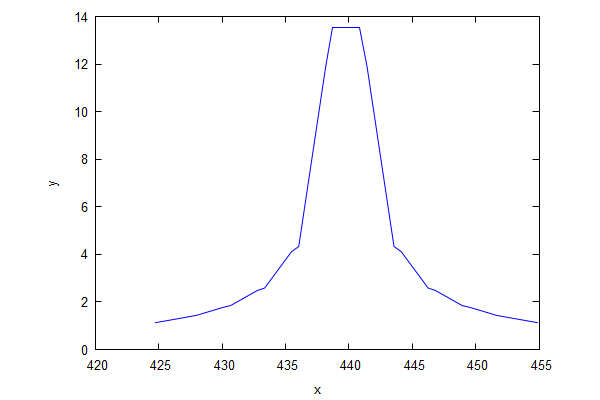Fig. 3. "Guessing" frequency 439.8 Hz. Looks better.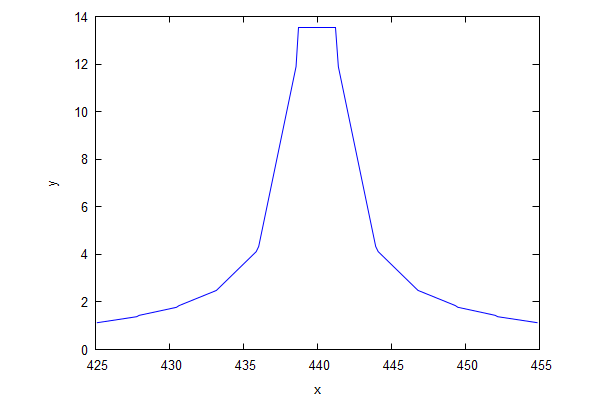Fig. 4. "Guessing" frequency 440 Hz. Looks about as smooth as we're going to get.

There are some considerable drawbacks to this approach. The biggest one is that I had to look at the results. The second drawback is that I don't have a number to put to these to decide which is better. And this is transformed from one of the simplest types of wave, a sine wave. To evaluate the soundness of the guess, I would need some formula to fit the points to.

## Friday, March 12, 2021

### Finding Frequency Fourier Style

Last week, I had a look at the FFT module in Maxima and it was fun times. I was a little disappointed at the interpolated result for the frequency when analyzing the results of the FFT of the 440 Hz waveform. Mind you, if the application is to find a nearest note frequency and, being a human with an at least average sense of musical perception, you already know you're hearing notes that sound like 12 semitones per octave (exclusive counting, i.e., C up to B), then you can coerce the results to the nearest value of  $$(440) 2^{i/12}$$, for $$i$$ over integers. If you don't intend to get any deeper than determining the major pitches, then this is fine. (Could get more tricky if the whole thing is internally shift tuned—everything a half of a semitone flat, but internally tuned, but then maybe you could create a filter to catch that case and accordingly shift your frequency base from 440 to something else that models the sound better.)

We got close enough last time for goal 1 (find the basic notes), but still, I wondered whether I could get closer to the true frequency than last time. I also thought I should use a different example frequency so nobody gets the wrong idea that this is somehow only good for integer frequencies. (Integers are discrete, but discreteness goes beyond just integers.)

So, let's use $$(440) 2^{1/12}$$ or approximately 466.16 Hz.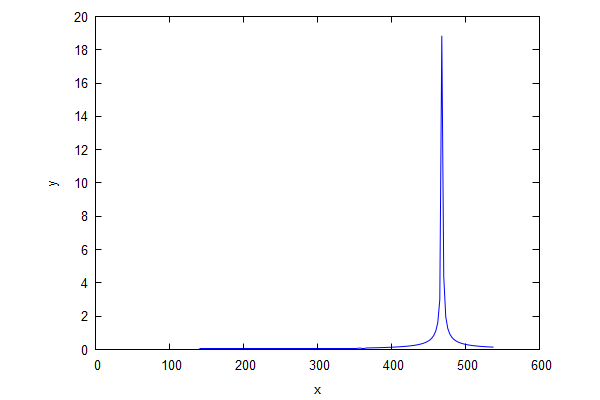Fig. 1. Frequency of 466.16 Hz.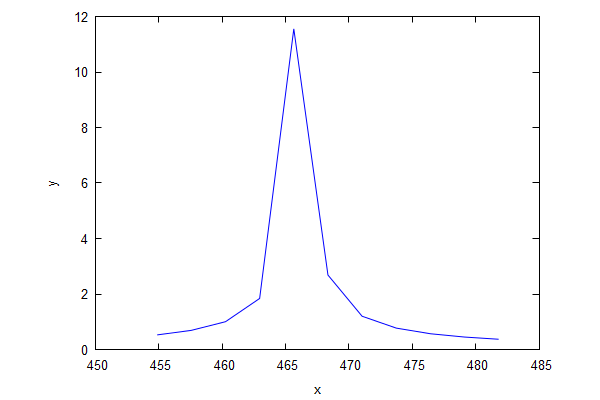Fig. 2. Zooming in a little closer on the points.

So, let's take these same points and try a cubic spline.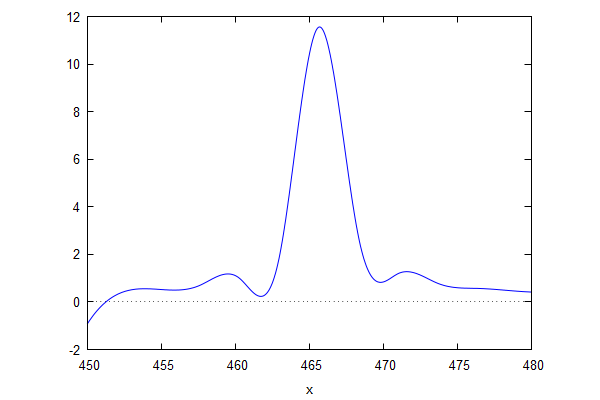Fig. 3. Cubic spline view of things. Still looks fake. But less fake in the middle.

The cubic spline is helpfully printed out with characteristic functions which are mainly readable. I pick out the one that has the interval of concern, namely, 0.5264689995542815*x^3-738.1894409400452*x^2+345013.3968874384*x-5.374983574143041*10^7. We can use differentiation to find the location of the maximum value.

Which gives roots of [x=465.6995765525049,x=469.0682718425062]. Clearly 465.7 is the one we want. We're not any closer than we were last time using linear interpolation, although the general appearance of the graph looks more realistic and we did include another bit of the Maxima library.

## Saturday, March 6, 2021

### FFT - Local Symmetry Inferred Peak

As I was thinking about the previous post, I thought there might be a way to estimate the location of the peak. That is, to find the location in between the discrete data points where the peak probably occurs.

I tried using realpart and imagpart and abs to see the differences and it seemed like realpart gives me the best view of the relative amplitudes when there are multiple frequencies involved. I also decided to apply an index shift since that seems to match the frequency better although I'm not satisfied with it technically yet.

Let's zoom in on the location of one of the peaks and see what it looks like:Fig. 1. Looks like we should be able to make a better guess than just picking one of the points.

Here are the actual points as frequency, absolute, real part value pairs:

[[427.972412109375,1.373307119161833],[430.6640625,1.783783923237471],[433.355712890625,2.525090138349324],[436.04736328125,4.273342049313825],[438.739013671875,13.47741003943344],[441.4306640625,11.94536977902466],[444.122314453125,4.166745114303215],[446.81396484375,2.532438127248608],[449.505615234375,1.822956914133206],[452.197265625,1.426082632315385],[454.888916015625,1.172306075942115]]

For a first crack at it, we might try linear interpolation on the two pairs of points on either side of the gap that contains the peak. Based on the Wikipedia article of the continuous version, we are certainly deviating from the shape of the real thing. Dauntless we press on to see what we get, because maybe we can live with a slight improvement that we know isn't perfect.

So, here we go do linear interpolation and find the line intersection using Maxima.

[[x=439.729058165623,y=16.86285595968834]]

Let's put that point in the middle and see what it looks like: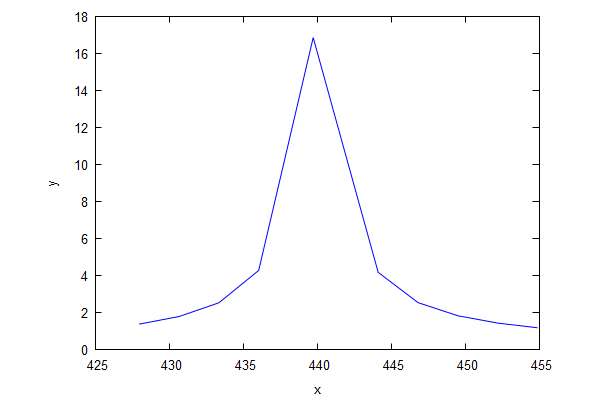Fig. 2. Hmm, closer, but looks kinda fake to me.

Somewhat predictably, this looks as fake as it really is. I think this graph makes it just how clear that linear interpolation is slightly bogus here. Not completely bogus though, it got us closer, right? So, we've ended up on the left side of the true peak which we know should happen exactly at 440 Hz. Why? The slope on the right side of the true peak is further away from the peak and therefore has a lower (absolute) slope value—it is less steep than it would be if it was closer to the peak. This is the weakness of linear interpolating this. We will end up closer to the higher of the two near-peak points than we should.

To get closer, we might want to try a different type of interpolation. Maybe a cubic spline?